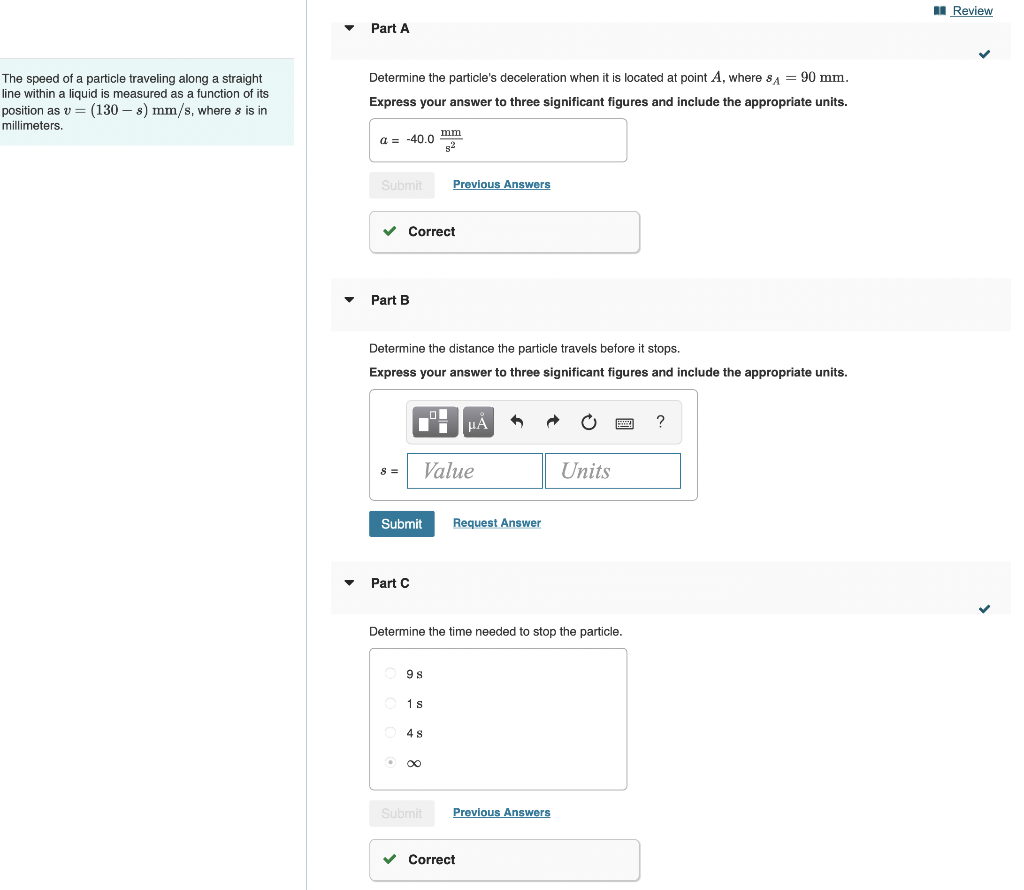# Question Solved1 AnswerNeed help with part B please write neat so I can understand. The speed of a particle traveling along a straight line within a liquid is measured as a function of its position as v = (130 s) mm/s, where s is in millimeters. Part A Determine the particle's deceleration when it is located at point A, where SA = 90 mm. Express your answer to three significant figures and include the appropriate units. a = -40.0 mm 5² Submit Previous Answers ✓ Correct Part B Determine the distance the particle travels before it stops. Express your answer to three significant figures and include the appropriate units. μÀ ? 8 = Value Units Submit Request Answer Part C Determine the time needed to stop the particle. 98 1s 4 s O ∞ Submit Previous Answers ✓ Correct Review5NBRK0 The Asker · Mechanical Engineering

Need help with part B please write neat so I can understand.Transcribed Image Text: The speed of a particle traveling along a straight line within a liquid is measured as a function of its position as v = (130 s) mm/s, where s is in millimeters. Part A Determine the particle's deceleration when it is located at point A, where SA = 90 mm. Express your answer to three significant figures and include the appropriate units. a = -40.0 mm 5² Submit Previous Answers ✓ Correct Part B Determine the distance the particle travels before it stops. Express your answer to three significant figures and include the appropriate units. μÀ ? 8 = Value Units Submit Request Answer Part C Determine the time needed to stop the particle. 98 1s 4 s O ∞ Submit Previous Answers ✓ Correct Review
More
Transcribed Image Text: The speed of a particle traveling along a straight line within a liquid is measured as a function of its position as v = (130 s) mm/s, where s is in millimeters. Part A Determine the particle's deceleration when it is located at point A, where SA = 90 mm. Express your answer to three significant figures and include the appropriate units. a = -40.0 mm 5² Submit Previous Answers ✓ Correct Part B Determine the distance the particle travels before it stops. Express your answer to three significant figures and include the appropriate units. μÀ ? 8 = Value Units Submit Request Answer Part C Determine the time needed to stop the particle. 98 1s 4 s O ∞ Submit Previous Answers ✓ Correct Review